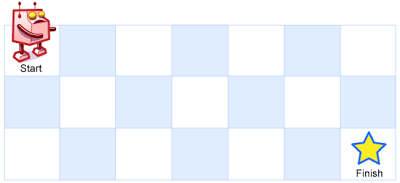#DAY 22
2
Software Development

## [Day 22] 從LeetCode學演算法 - 0062. Unique Paths (Medium)

``````Question:
A robot is located at the top-left corner of a m x n grid (marked ‘Start’ in the diagram below).
The robot can only move either down or right at any point in time. The robot is trying to reach the bottom-right corner of the grid (marked ‘Finish’ in the diagram below).
How many possible unique paths are there?
````````````Above is a 7 x 3 grid. How many possible unique paths are there?
Note: m and n will be at most 100.
``````
``````Example 1:
Input: m = 3, n = 2
Output: 3
Explanation:
From the top-left corner, there are a total of 3 ways to reach the bottom-right corner:
1. Right -> Right -> Down
2. Right -> Down -> Right
3. Down -> Right -> Right
``````
``````Example 2:
Input: m = 7, n = 3
Output: 28
``````

1. 機器人所站的原點 (按我們的定義一開始就在此，故可以將方法數定為1)
2. 最左邊的一排格子 (僅取決於走到其上面格子的方法數)
3. 最上面的一排格子 (僅取決於走到其左邊格子的方法數)

(註:由於這邊row跟column的交換並不影響結果，

Java:

``````class Solution {
public int uniquePaths(int m, int n) {
int[][] dp = new int[m][n];
for (int i = 0; i < m; i++) dp[i] = 1;
for (int i = 0; i < n; i++) dp[i] = 1;

for (int i = 1; i < m; i++) {
for (int j = 1; j < n; j++) {
dp[i][j] = dp[i - 1][j] + dp[i][j - 1];
}
}
return dp[m - 1][n - 1];
}
}
``````

Python這邊收尾的時候可以用dp[-1][-1]來表示尾端的元素，

Python:

``````class Solution:
def uniquePaths(self, m: int, n: int) -> int:
dp = [[0 for i in range(n)] for j in range(m)]
for i in range(m):
dp[i] = 1
for i in range(n):
dp[i] = 1

for i in range(1, m):
for j in range(1, n):
dp[i][j] = dp[i-1][j] + dp[i][j-1]

return dp[-1][-1]
``````

「時間/空間複雜度？」
(均為O(m * n))

「空間複雜度是否可以降低？」
(理論上可以，因為實際每次處理的關連部分僅有兩行或兩列，

「是否有更簡單的解？」
(DP的話，沒有，數學的話，有。

0169. Majority Element (Easy)